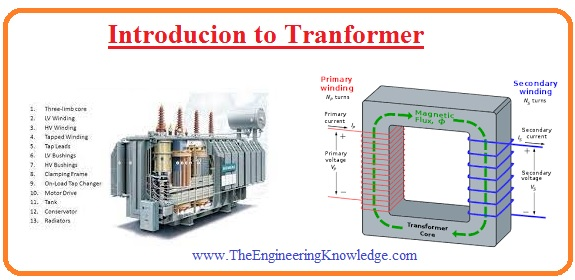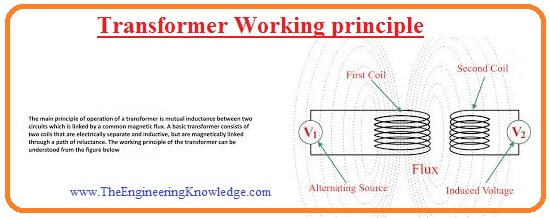Hello, friends welcome to the learning platform. In this post, we will have a detailed look at Introduction to Transformer. There are numerous devices used in our electrical power system such as a motor-generator that are further divided into the ac motor dc motor similar ac generator ac motor. The work of motor transforms the electrical power to mechanical power and generator transform mechanical power to electrical power.

There is such device used in the power system that not generate and transforms power but it varies the voltage level from one to other that called transformer. In other words, the value of voltage at its input will be different from the value of the voltage at its output. In this post, we will define its working, applications construction, and some other parts with the details. So let’s get started with Introduction to Transformer.

## Introducion to Tranformer

• The transformer is an electrical instrument that used to vary the voltage level from one to another with the use of a magnetic field.
• If the voltage level is decreasing at the output is called step down and if increases called to step-up transformer.
• There are 2 or more than 2 windings are wounded at a single core of ferromagnetic material.
• there is no physical link between these two windings but are linked magnetically.
• One winding of a transformer is linked to the input supply and the other is linked to the output or load.
• The windings of transformer that is attached to the input is called primary and other called secondary.
• If in certain cases there is 3rd windings is used that known as tertiary windings.
• The working phenomena of transformer depend on the Faraday Law that says the rate of change of flux induces the voltage in the conductor where the flux is varying.### Transformer Working principle

• The working principle of transformer is followed the mutual induction that says change of flux link to other windings or conductor material induces voltages.
• As there is 2 windings in the transformer when ac supply is given to the input or primary windings then due to supply flux is produced in the windings.
• The flux induced in the primary windings also linked to the second windings through passing the core of the transformer and voltage induces in the secondary windings.
• The value of voltage induced at secondary windings is dependent on the number of turns at secondary windings.
• There is some part of flux which not crosses the secondary winding is known as leakage flux
• The induced voltage is also followed the Faraday law. The quantity of voltage induced relies on the number of turns windings at respective sides.

V1/V2 = N1/N2### Construction of transformer

• The main parts of the transformer are discussed below.Transformer Core

• The core is constructed with the substance has a high value of permeability it means this material offers such path that has less value of reluctance to flow of flux from primary to secondary.
• The core normally designed in the form of square or rectangular according to requirements.
• If the transformer core is created with the ferromagnetic material like iron then air a large amount of flux flow from one winding to other due to less flux leakage.
• The core is created through silicon and has lamination. the lamination helps to reduce the eddy current.
• The thickness of lamination is about 0.35 to five millimeters.

Tranfsforemr Conservator

• In this part of the transformer, there is the conservation of oil. It is a cylinder-like configuration that has and placed at the upper portion of the transformer.
• The oil level in this part is mid so that when it expands due to temperature rises to the upper level.

Transformer Winding

• Normally 2 windings exist in the transformer firs one is primary where input is linked and 2nd one secondary where output is taken..
• The windings of the transformer is created through the use of copper that is high level.
• There is insulation is rapped at the conductors through which the current is passing.
• the voltage given to the primary windings is known as primary voltage and in a results voltage produced at secondary windings is secondary voltage.
• So voltage named as LV and HV according to a voltage level.

## Transformer type

Step-up transformer

• In this category of the transformer, there is less number of turns at a primary and a large number of turns at secondary.
• This configuration of the transformer helps to increase the voltage level at the secondary side than the primary side.

Step Down transformer

• In this type of transformer, there is a large number of turns at primary and less number of turns at secondary.
• The voltage value of secondary is less than primary so it prefers such application where less voltage is needed

Core Type Transforemr

• In this category of transformer windings is wounded in such configuration that it covers the core or can say the core is outer side.
• Normally this type of arrangement used a distribution transformer.
• The cylinder shape windings are configured in this type of transformer and windings shape can be rectangle, or circular.
• The small size transformer uses a rectangle shape core.

Shell Type of Transformer

• In this type of transformer, the core is at the outer side and the winding is wound at the inner side.
• In this transformer 2 windings are positioned at one arm of the core. The configuration of windings is like the multiple-layer disc.
• To make separation and avoid layers from practical connection there is insulation is wrapped.

That is a detailed post about Transformer if you have any further queries mentioned in the comments. See you in the next post have a nice day.